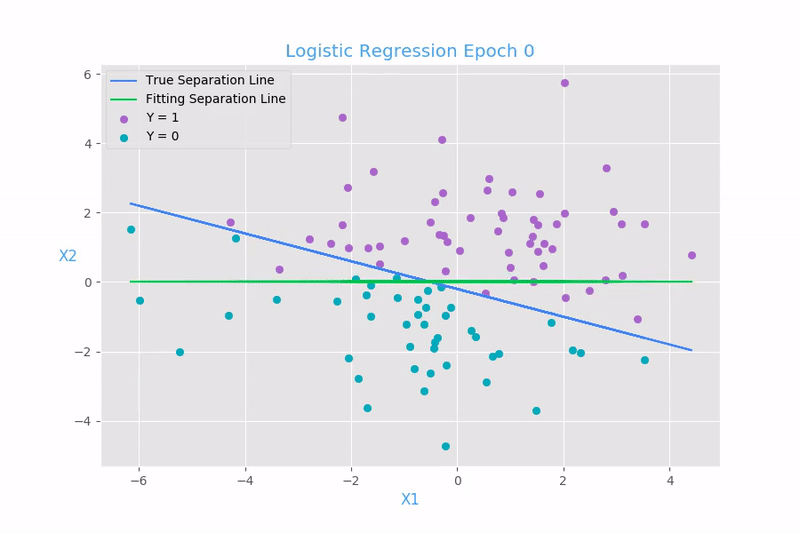# How To Build A Simple Neural Network From Scratch

A a while ago, I transitioned from Android development into deep learning. I went into the reason on why I did it in this article, but the gist of it is that I needed something more technically and with less graphic design.

Deep learning is based upon the concept of a neural network. There are different types of neural networks, but we’re not going to go into that in this article.

In this article, I am going to show you how to build a simple neural network in Python. The only dependency that we are going to need in order to build the network is numpy. If you don’t already know, numpy is a Python library which makes performing math very easy. You could do this using native Python, but why would you do that. If you want to go deeper and understand what some of the numpy functions do, don’t go and build your own functions which do the exact same thing. Just look up the math behind the function.

Here, we define the NeuralNetwork class and write our __init__ function.

In the __init__ function, we seed the random number generator. We do this so that it generates the same numbers each time. This makes debugging easier.

Next we define the weights of the connections. This is really a perceptron more than a neural network. A perceptron is consisted only of a input and an output layer. It’s just a really simple neural network, really consisting of a single neuron.The image above shows a diagram of a perceptron. As you can see, the weights are the values on the connections to the neuron.

Now comes the fun part, the training.

### Training

This part is accomplished by using a procedure called gradient descent.Gradient descent is an iterative optimization algorithm used for finding the local minima of a function. This function is basically used to find the separation point between two values. So using this algorithm you could write a script which determines if a person is a male or a female based upon a couple of their physical attributes (weight, height, shoe size, age etc.), but that kind of thing would be considered insensitive and sexist these days.

We’ll repeat this functions n times, where n is the selected number of iterations.

We calculate the error. The error is the difference between the output between the output we predicted and the true value. We’ll define the predict function in a minute here.

Next we adjust our weights. we do that by calculating the dot product of the transpose of the input matrix and the product of error and the sigmoid derivative of the predicted output (we’ll define that function in a minute as well). And then just add the adjustment to the weight.

This is the derivative of the sigmoid function. We’ll need to define the sigmoid function as well.

The sigmoid function takes a value, normalises it and puts it in a range between 0 and 1. It defines an s-shaped curve like this:### Predicting

Now we’ll need to predict the output.

We return value returned by the sigmoid function which has received the dot product of the inputs and the weights.

Since we’ll be passing values of 0s and 1s as inputs and outputs into the neural network, the predict function we’ll return a number between 0 and 1.

Because of that, this script will only be useful for predicting something which only can have two outputs. The more training data we give to the neural network, the more accurate it will be at predicting the output.

### Define the main function

This part is pretty straight forward. We create some training data. The training inputs do not have to be 0s and 1s, they can be any values you wish, but the training outputs do need to be either 0 or 1. We cover cases where you have more than two possible outputs in a future article.